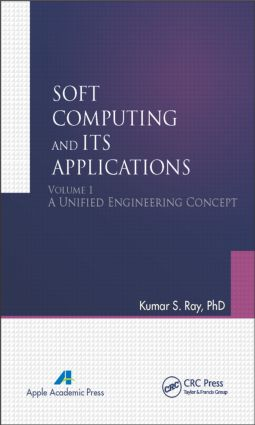# Soft Computing and Its Applications, Volume One

## A Unified Engineering Concept, 1st Edition

632 pages | 198 B/W Illus.

##### Purchasing Options:\$ = USD
Hardback: 9781926895383
pub: 2014-09-16
\$220.00
x
eBook (VitalSource) : 9780429071980
pub: 2014-09-16
from \$28.98

FREE Standard Shipping!

### Description

This is volume 1 of the two-volume set Soft Computing and Its Applications. This volume explains the primary tools of soft computing as well as provides an abundance of working examples and detailed design studies. The book starts with coverage of fuzzy sets and fuzzy logic and their various approaches to fuzzy reasoning. Precisely speaking, this book provides a platform for handling different kinds of uncertainties of real-life problems. It introduces the reader to the topic of rough sets. This book’s companion volume, Volume 2: Fuzzy Reasoning and Fuzzy Control, will move forward from here to discuss several advanced features of soft computing and application methodologies.

This new book:

• Discusses the present state of art of soft computing

•Includes the existing application areas of soft computing

• Presents original research contributions

• Discusses the future scope of work in soft computing

The book is unique in that it bridges the gap between theory and practice, and it presents several experimental results on synthetic data and real-life data. The book provides a unified platform for applied scientists and engineers in different fields and industries for the application of soft computing tools in many diverse domains of engineering.

### Reviews

"This two-volume textbook set is a quite elementary, but rather comprehensive, introduction to the field of soft computing, accessible not only for undergraduates in mathematics, but also for students in computer science and engineering. The presentation is essentially correct, offers figures for most of the notions it defines, and presents lots of detailed numerical examples. Volume 1 starts with an explanation of the notion of soft computing and continues with chapters on fuzzy sets, fuzzy operators, fuzzy relations, fuzzy logic, fuzzy implications, fuzzy if-then models, and rough sets. Volume 2 covers in separate chapters the topics of fuzzy reasoning, fuzzy reasoning based on the concept of similarity, and fuzzy control."

—Siegfried J. Gottwald, writing in Zentralblatt MATH, 1308

Notion of Soft Computing

1.1. Introduction

1.2. Scope for future work

Fuzzy Sets, Fuzzy Operators and Fuzzy Relations

2.1. Introduction

2.2. Fuzzy set

2.3. Metrics for fuzzy numbers

2.4. Difference in fuzzy set

2.5. Distance in fuzzy set

2.6. Cartesian product of fuzzy set

2.7. Operators on fuzzy set

2.8. Other operations in fuzzy set

2.9. Geometric interpretation of fuzzy sets

2.10. T-operators

2.11. Aggregation operators

2.12. Probability versus Possibility

2.13. Fuzzy event

2.14. Uncertainty

2.15. Measure of fuzziness

2.16. Type-2 fuzzy sets

2.17. Relation

Fuzzy Logic

3.1. Introduction

3.2. Preliminaries of logic

3.3. Lukasiewicz logic

3.4. Fuzzy logic

3.5. Fuzzy logic as viewed by Zadeh

3.6. Algebric structure in fuzzy logic

3.7. Critical appreciations on fuzzy logic

3.8. Generating logic for fuzzy set

3.9. Fuzzifying non-classical logics

3.10. Bridging the gap between fuzzy logic and quantum logic

3.11. Futuristic ambitions of fuzzy logic

Fuzzy Implications and Fuzzy If-Then Models

4.1. Introduction

4.2. Syntax and semantics of material implication

4.3. Fuzzy modifiers (hedges)

4.4. Linguistic truth value

4.5. Group decision making based on linguistic decision process

4.6. Linguistic assessments and combination of linguistic values

4.7. Linguistic preference relations and linguistic choice process

4.8. Fuzzy systems as function approximators

4.9. Extracting fuzzy rules from sample data points

4.10. Fuzzy basis functions

4.11. Extracting fuzzy rules from clustering of training samples

4.12. Representation of fuzzy IF-THEN rules by petri net

4.13. Transformations among various rule based fuzzy models

4.14. Losless rule reduction techniques for fuzzy system

4.15. Simplification of fuzzy rule base using similarity measure

4.16. Qualitative modeling based on fuzzy logic

Rough Set

5.1. Introduction

5.2. Gateway to roughset concept

5.3. Approximation spaces and set approximation

5.4. Rough membership function

5.5. Information systems

5.6. Indiscernibility relation

5.7. Some further illustration on set approximation

5.8. Dependency of attributes

5.9. Approximation and accuracy of classification

5.10. Reduction of attributes

5.11. Discernibility matrices and functions

5.12. Significance of attributes and approximate reducts

5.13. Decision rule synthesis

5.14. Case study: diagnosis of dengue based on rough set concept

5.15. Rough sets, Bayes’ rule & multivalued logic

5.16. Rough sets and data mining

Index

### About the Author

Kumar S. Ray, PhD, is a professor in the Electronics and Communication Science Unit at the Indian Statistical Institute, Kolkata, India. He has written a number of articles published in international journals and has presented at several professional meetings. His current research interests include artificial intelligence, computer vision, commonsense reasoning, soft computing, non-monotonic deductive database systems, and DNA computing.

### Subject Categories

##### BISAC Subject Codes/Headings:
COM037000
COMPUTERS / Machine Theory